# PHP-Overview.ppt

28. May 2023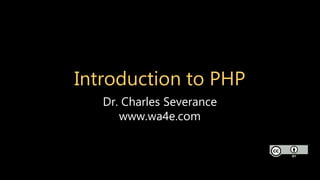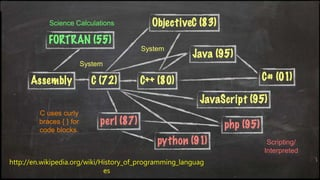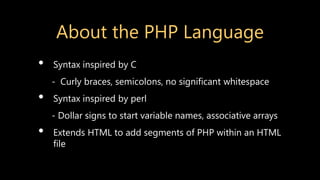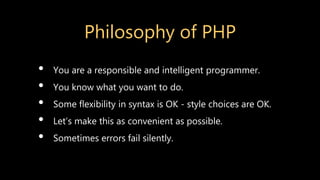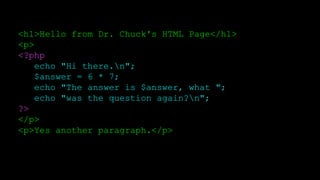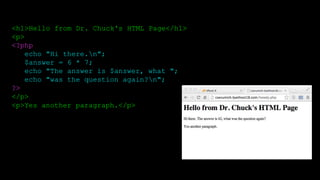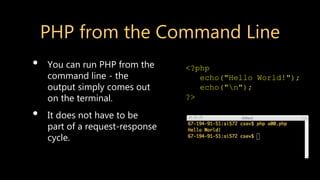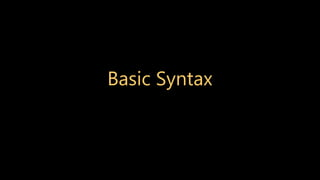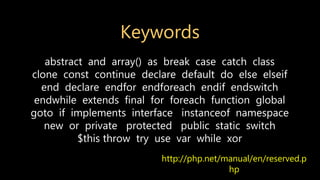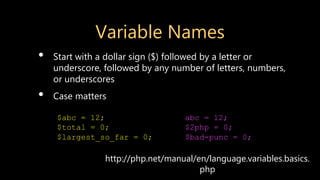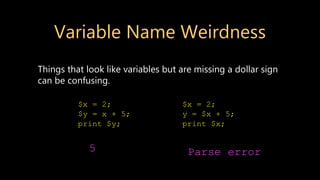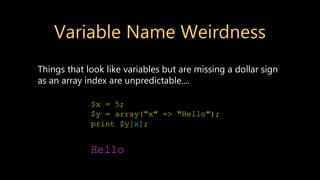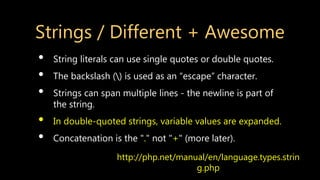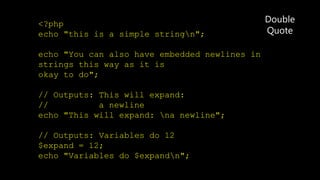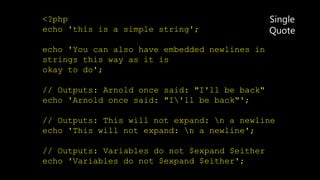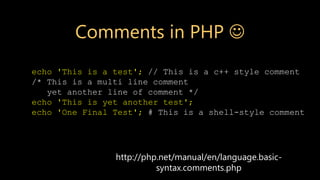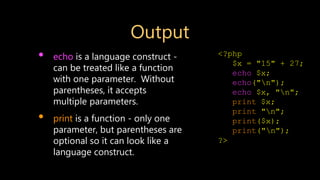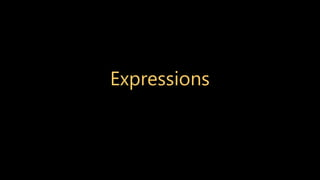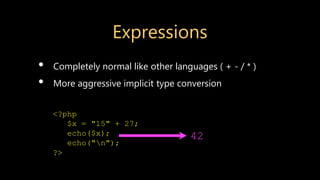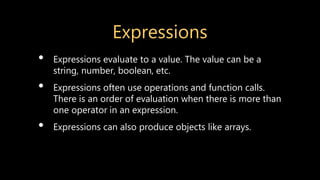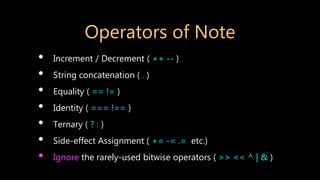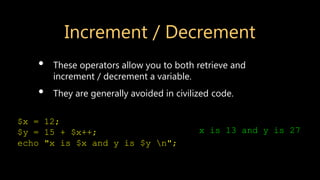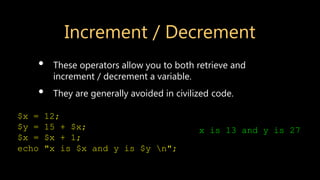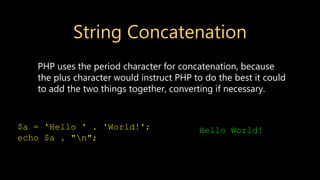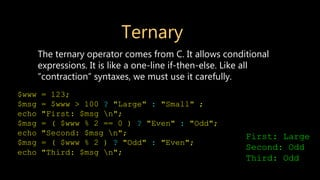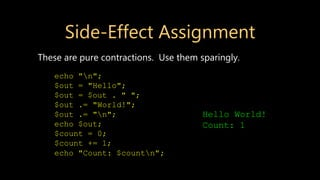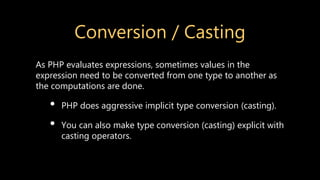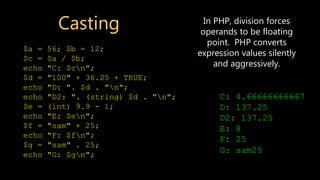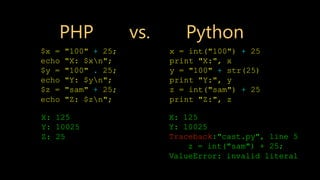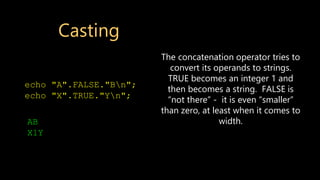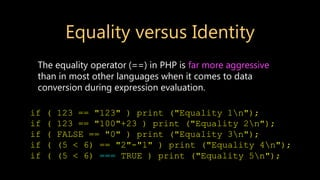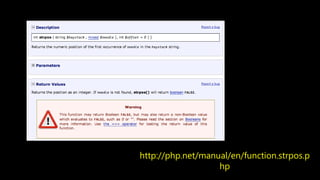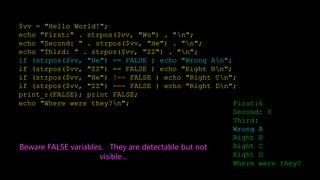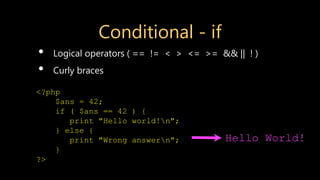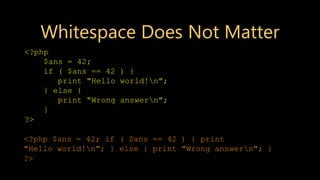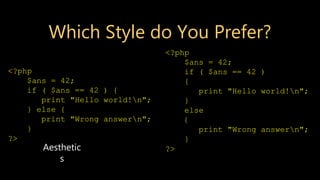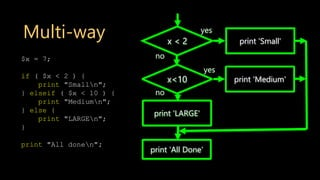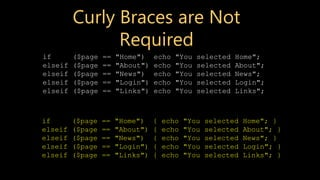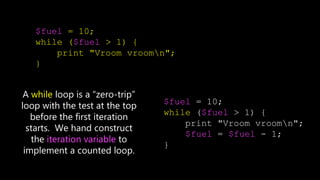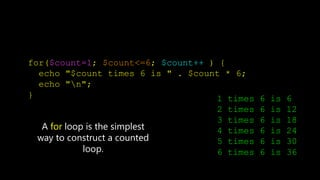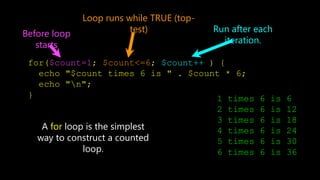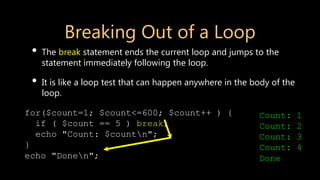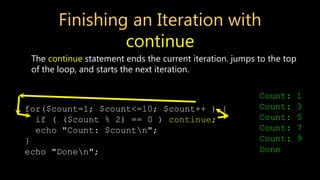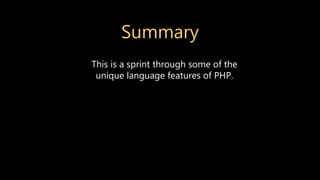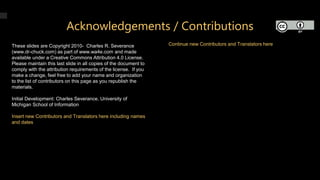1 von 47

### PHP-Overview.ppt

• 1. Introduction to PHP Dr. Charles Severance www.wa4e.com
• 3. About the PHP Language • Syntax inspired by C - Curly braces, semicolons, no significant whitespace • Syntax inspired by perl - Dollar signs to start variable names, associative arrays • Extends HTML to add segments of PHP within an HTML file
• 4. Philosophy of PHP • You are a responsible and intelligent programmer. • You know what you want to do. • Some flexibility in syntax is OK - style choices are OK. • Let’s make this as convenient as possible. • Sometimes errors fail silently.
• 5. <h1>Hello from Dr. Chuck's HTML Page</h1> <p> <?php echo "Hi there.n"; \$answer = 6 * 7; echo "The answer is \$answer, what "; echo "was the question again?n"; ?> </p> <p>Yes another paragraph.</p>
• 6. <h1>Hello from Dr. Chuck's HTML Page</h1> <p> <?php echo "Hi there.n"; \$answer = 6 * 7; echo "The answer is \$answer, what "; echo "was the question again?n"; ?> </p> <p>Yes another paragraph.</p>
• 7. PHP from the Command Line • You can run PHP from the command line - the output simply comes out on the terminal. • It does not have to be part of a request-response cycle. <?php echo("Hello World!"); echo("n"); ?>
• 9. Keywords http://php.net/manual/en/reserved.p hp abstract and array() as break case catch class clone const continue declare default do else elseif end declare endfor endforeach endif endswitch endwhile extends final for foreach function global goto if implements interface instanceof namespace new or private protected public static switch \$this throw try use var while xor
• 10. Variable Names • Start with a dollar sign (\$) followed by a letter or underscore, followed by any number of letters, numbers, or underscores • Case matters http://php.net/manual/en/language.variables.basics. php \$abc = 12; \$total = 0; \$largest_so_far = 0; abc = 12; \$2php = 0; \$bad-punc = 0;
• 11. Variable Name Weirdness Things that look like variables but are missing a dollar sign can be confusing. \$x = 2; \$y = x + 5; print \$y; \$x = 2; y = \$x + 5; print \$x; 5 Parse error
• 12. Variable Name Weirdness Things that look like variables but are missing a dollar sign as an array index are unpredictable.... \$x = 5; \$y = array("x" => "Hello"); print \$y[x]; Hello
• 13. Strings / Different + Awesome • String literals can use single quotes or double quotes. • The backslash () is used as an “escape” character. • Strings can span multiple lines - the newline is part of the string. • In double-quoted strings, variable values are expanded. • Concatenation is the "." not "+" (more later). http://php.net/manual/en/language.types.strin g.php
• 14. <?php echo "this is a simple stringn"; echo "You can also have embedded newlines in strings this way as it is okay to do"; // Outputs: This will expand: // a newline echo "This will expand: na newline"; // Outputs: Variables do 12 \$expand = 12; echo "Variables do \$expandn"; Double Quote
• 15. <?php echo 'this is a simple string'; echo 'You can also have embedded newlines in strings this way as it is okay to do'; // Outputs: Arnold once said: "I'll be back" echo 'Arnold once said: "I'll be back"'; // Outputs: This will not expand: n a newline echo 'This will not expand: n a newline'; // Outputs: Variables do not \$expand \$either echo 'Variables do not \$expand \$either'; Single Quote
• 16. http://php.net/manual/en/language.basic- syntax.comments.php echo 'This is a test'; // This is a c++ style comment /* This is a multi line comment yet another line of comment */ echo 'This is yet another test'; echo 'One Final Test'; # This is a shell-style comment Comments in PHP 
• 17. Output • echo is a language construct - can be treated like a function with one parameter. Without parentheses, it accepts multiple parameters. • print is a function - only one parameter, but parentheses are optional so it can look like a language construct. <?php \$x = "15" + 27; echo \$x; echo("n"); echo \$x, "n"; print \$x; print "n"; print(\$x); print("n"); ?>
• 19. Expressions • Completely normal like other languages ( + - / * ) • More aggressive implicit type conversion <?php \$x = "15" + 27; echo(\$x); echo("n"); ?> 42
• 20. Expressions • Expressions evaluate to a value. The value can be a string, number, boolean, etc. • Expressions often use operations and function calls. There is an order of evaluation when there is more than one operator in an expression. • Expressions can also produce objects like arrays.
• 21. Operators of Note • Increment / Decrement ( ++ -- ) • String concatenation ( . ) • Equality ( == != ) • Identity ( === !== ) • Ternary ( ? : ) • Side-effect Assignment ( += -= .= etc.) • Ignore the rarely-used bitwise operators ( >> << ^ | & )
• 22. Increment / Decrement • These operators allow you to both retrieve and increment / decrement a variable. • They are generally avoided in civilized code. \$x = 12; \$y = 15 + \$x++; echo "x is \$x and y is \$y n"; x is 13 and y is 27
• 23. Increment / Decrement • These operators allow you to both retrieve and increment / decrement a variable. • They are generally avoided in civilized code. \$x = 12; \$y = 15 + \$x; \$x = \$x + 1; echo "x is \$x and y is \$y n"; x is 13 and y is 27
• 24. String Concatenation PHP uses the period character for concatenation, because the plus character would instruct PHP to do the best it could to add the two things together, converting if necessary. \$a = 'Hello ' . 'World!'; echo \$a . "n"; Hello World!
• 25. Ternary The ternary operator comes from C. It allows conditional expressions. It is like a one-line if-then-else. Like all “contraction” syntaxes, we must use it carefully. \$www = 123; \$msg = \$www > 100 ? "Large" : "Small" ; echo "First: \$msg n"; \$msg = ( \$www % 2 == 0 ) ? "Even" : "Odd"; echo "Second: \$msg n"; \$msg = ( \$www % 2 ) ? "Odd" : "Even"; echo "Third: \$msg n"; First: Large Second: Odd Third: Odd
• 26. Side-Effect Assignment These are pure contractions. Use them sparingly. echo "n"; \$out = "Hello"; \$out = \$out . " "; \$out .= "World!"; \$out .= "n"; echo \$out; \$count = 0; \$count += 1; echo "Count: \$countn"; Hello World! Count: 1
• 27. Conversion / Casting As PHP evaluates expressions, sometimes values in the expression need to be converted from one type to another as the computations are done. • PHP does aggressive implicit type conversion (casting). • You can also make type conversion (casting) explicit with casting operators.
• 28. Casting \$a = 56; \$b = 12; \$c = \$a / \$b; echo "C: \$cn"; \$d = "100" + 36.25 + TRUE; echo "D: ". \$d . "n"; echo "D2: ". (string) \$d . "n"; \$e = (int) 9.9 - 1; echo "E: \$en"; \$f = "sam" + 25; echo "F: \$fn"; \$g = "sam" . 25; echo "G: \$gn"; C: 4.66666666667 D: 137.25 D2: 137.25 E: 8 F: 25 G: sam25 In PHP, division forces operands to be floating point. PHP converts expression values silently and aggressively.
• 29. PHP vs. Python \$x = "100" + 25; echo "X: \$xn"; \$y = "100" . 25; echo "Y: \$yn"; \$z = "sam" + 25; echo "Z: \$zn"; X: 125 Y: 10025 Z: 25 x = int("100") + 25 print "X:", x y = "100" + str(25) print "Y:", y z = int("sam") + 25 print "Z:", z X: 125 Y: 10025 Traceback:"cast.py", line 5 z = int("sam") + 25; ValueError: invalid literal
• 30. Casting echo "A".FALSE."Bn"; echo "X".TRUE."Yn"; AB X1Y The concatenation operator tries to convert its operands to strings. TRUE becomes an integer 1 and then becomes a string. FALSE is “not there” - it is even “smaller” than zero, at least when it comes to width.
• 31. Equality versus Identity The equality operator (==) in PHP is far more aggressive than in most other languages when it comes to data conversion during expression evaluation. if ( 123 == "123" ) print ("Equality 1n"); if ( 123 == "100"+23 ) print ("Equality 2n"); if ( FALSE == "0" ) print ("Equality 3n"); if ( (5 < 6) == "2"-"1" ) print ("Equality 4n"); if ( (5 < 6) === TRUE ) print ("Equality 5n");
• 33. \$vv = "Hello World!"; echo "First:" . strpos(\$vv, "Wo") . "n"; echo "Second: " . strpos(\$vv, "He") . "n"; echo "Third: " . strpos(\$vv, "ZZ") . "n"; if (strpos(\$vv, "He") == FALSE ) echo "Wrong An"; if (strpos(\$vv, "ZZ") == FALSE ) echo "Right Bn"; if (strpos(\$vv, "He") !== FALSE ) echo "Right Cn"; if (strpos(\$vv, "ZZ") === FALSE ) echo "Right Dn"; print_r(FALSE); print FALSE; echo "Where were they?n"; First:6 Second: 0 Third: Wrong A Right B Right C Right D Where were they? Beware FALSE variables. They are detectable but not visible...
• 35. Conditional - if • Logical operators ( == != < > <= >= && || ! ) • Curly braces <?php \$ans = 42; if ( \$ans == 42 ) { print "Hello world!n"; } else { print "Wrong answern"; } ?> Hello World!
• 36. Whitespace Does Not Matter <?php \$ans = 42; if ( \$ans == 42 ) { print "Hello world!n"; } else { print "Wrong answern"; } ?> <?php \$ans = 42; if ( \$ans == 42 ) { print "Hello world!n"; } else { print "Wrong answern"; } ?>
• 37. Which Style do You Prefer? <?php \$ans = 42; if ( \$ans == 42 ) { print "Hello world!n"; } else { print "Wrong answern"; } ?> <?php \$ans = 42; if ( \$ans == 42 ) { print "Hello world!n"; } else { print "Wrong answern"; } ?> Aesthetic s
• 38. Multi-way \$x = 7; if ( \$x < 2 ) { print "Smalln"; } elseif ( \$x < 10 ) { print "Mediumn"; } else { print "LARGEn"; } print "All donen"; x < 2 print 'Small' yes no print 'All Done' x<10 print 'Medium' yes print 'LARGE' no